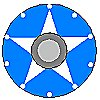# Shufflix: Statistical Validation

Assorted statistical tests are run on output from Shufflix.  The purpose is to test whether the hands generated are indeed unbiased.

### General Description of the Test Method Used

160 000 boards have been dealt offline using Shufflix's dealing algorithm.  This was accomplished by dealing 4000 runs of 40 boards each.  Each run uses the following input:
• 128 bits of random data acquired from www.random.org.  This fills the role of the entropy data gathered from /dev/urandom in the on-line verison of Shufflix.
• A systematically generated tournament name.
• The date 2001-12-31.
• The timestamp of the actual run of the dealing.
These 160 000 boards are then used to count significant statistics that are relevant from a bridge player's point of view.  Those statistics are compared with the theoretical distribution using the chi2 test.

The chi2 test yields a single number between zero and one, which can be taken as sort of measurement of how well the observations fit with the theoretically predicted results.   Large numbers (close to 1) denote a good fit while small numbers (close to 0) denote a bad fit.  A statistical test like this is often taken to be accepted at the 95% confidence level if the chi2 number is greater than 0.05.  Note, however, that the distribution of the chi2 statistic follows a uniform distribution between 0 and 1, so that one true result out of 20 is expected to be less than 0.05.

The statistical tests can be criticized for using one sample to generate several different statistics, since these different observations cannot be completely independent of each other.  For the time being, that will not be helped.

### Results

 Test name Test description chi2 statistic observations spreadsheet n-pat Pattern of North's hand, e.g. 5-5-2-1, 4-4-4-1.   4-4-3-2 is most likely. 0.29 n-pat.txt n-pat.xls d7-patt Taking groups of four consecutive boards, who gets the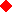7, e.g. NNSW, EWEW.  All patterns are equally likely. 0.84 d7-patt.txt d7-patt.xls hcp How many high card points does a randomly selected hand on each board have, e.g. 16 hcp, 3 hcp.  10 hcp is the most likely outcome. 0.83 hcp.txt hcp.xls 8-card Number of 8-cards suits held by West per 1000 hands.  The typical value is around 4. 0.73 8-card.txt 8-card.xls void-hst Number of boards with a void per runs of 36 boards.  The typical value is 6 or 7. void-hst.txt sa-c2 Who gets the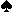A and the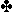2, e.g. E-E or N-S.  All 16 combinations are about equally likely, but combinations where the same hand gets both are a little less likely than those where different hands are involved. 0.26 sa-c2.txt sa-c2.xls di-acekg Who gets theA and theK?  This is basically the same kind of test as above. 0.76 di-acekg.txt di-acekg.xls h-akq Who gets the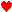K when theAQJ are on the same hand? 0.67 h-aqj.txt h-aqj.xls split When NS have exactly 8s between them, how do the remaining 5s split? 0.20 split.txt split.xls

The results are fine so far.  Empty chi2 values are given where the spreadsheet for the calculation is not made yet.  More statistics will appear later as they get processed.

Version 2001-09-08 / jbc